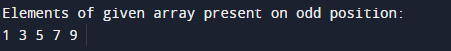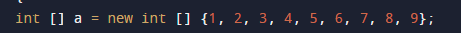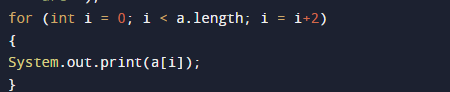# Java Program to print elements of an array present on odd position

In this tutorial you will learn about the Java Program to print elements of an array present on odd position and its application with practical example.

In this tutorial, we will learn to create a Java Program to print elements of an array present on odd position using java programming.

## Prerequisites

Before starting with this tutorial, we assume that you are best aware of the following Java programming concepts:

• Java Operators.
• Basic Input and Output function in Java.
• Class and Object in Java.
• Basic Java programming.
• If-else statements in Java.
• For loop in Java.

## What is Odd even Place in array ?

Odd-Even positions in an array are those element present within even index and for odd present in odd index are called odd even place as shown in the image below. So element on 1,3,5 are odd index value which is => 20,40 and 60 are odd index values.## Java Program to print elements of an array present on odd position

In this program we will print element in present in odd position within the array using for loop. We would first declared and initialized the required variables. Next, we will find odd place  element in an array. Lets have a look at the code.

## OutputIn the above program, we have first declared and initialized a set variables required in the program.

• a[]= it will hold array values
• i = for iteration.

After declaring variables we initiate values in  an array[]Algorithm

• 1: Start.
• 2: Initialize array with vales a[] = {1, 2, 3, 4, 5, 6, 7, 8, 9}
• 3: Simply print “elements of given array present at odd positions”.
• 4: Repeat  this step for(i=0; i< arr.length; i= i+2) unitll we  reach at last position of an array.
• 5: Print a[i].
•  6: End.with the help of for loop traverse through the array and printing elements of an array by incrementing value of i by i+2 till the last element of the array.

In this tutorial we have learn about the Java Program to print elements of an array present on odd position and its application with practical example. I hope you will like this tutorial.